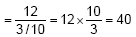Newbie

# A copper wire, 3 mm in diameter, is wound about a cylinder whose length is 12 cm, and diameter 10 cm, so as to cover the curved surface of the cylinder. Find the length and mass of the wire, assuming the density of copper to be 8.88 g per cm3. Q.1

• 0

How i solve this important question of ncert class 1oth chapter surfaces areas and volumes. Give me the easiest and simplest way to solve the exercise 13.5 question number 1 . Also give me the best solution of this question.A copper wire, 3 mm in diameter, is wound about a cylinder whose length is 12 cm, and diameter 10 cm, so as to cover the curved surface of the cylinder. Find the length and mass of the wire, assuming the density of copper to be 8.88 g per cm3.

Share

1. Given that,

Diameter of cylinder = 10 cm

So, radius of the cylinder (r) = 10/2 cm = 5 cm

∴ Length of wire in completely one round = 2πr = 3.14×5 cm = 31.4 cm

It is given that diameter of wire = 3 mm = 3/10 cm

∴ The thickness of cylinder covered in one round = 3/10 m

Hence, the number of turns (rounds) of the wire to cover 12 cm will be-Now, the length of wire required to cover the whole surface = length of wire required to complete 40 rounds

40 x 31.4 cm = 1256 cm

Radius of the wire = 0.3/2 = 0.15 cm

Volume of wire = Area of cross-section of wire × Length of wire

= π(0.15)2×1257.14

= 88.898 cm3

We know,

Mass = Volume × Density

= 88.898×8.88

= 789.41 gm

• 0